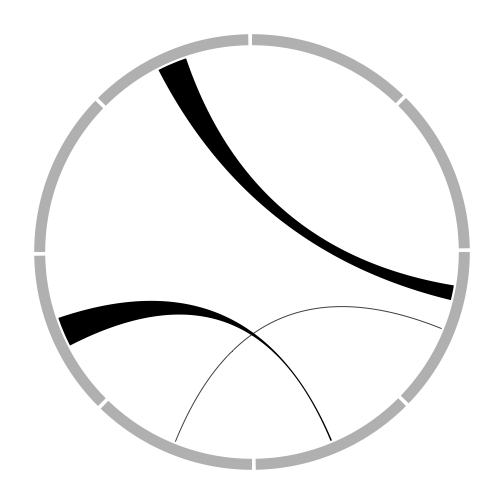## March 30, 2014

### Try circlize package

Today I try to circlize package. This package enables us to make Circos plot. Circos plot is well known for describing transitions of population. Also in Japan, the plot is used in the special TV program of the last election (below).

http://dl.dropboxusercontent.com/u/956851/test000002.jpg

circulize package has a good vignette, so I highly recommend you read that.

At this post I try just one example. In future articles, I will describe how to plot in more detail.

``````library(circlize)
par(mar = c(1, 1, 1, 1))
factors = letters[1:8]
circos.par(points.overflow.warning = FALSE)

# initialize
circos.initialize(factors = factors, xlim = c(0, 10))
circos.trackPlotRegion(factors = factors, ylim = c(0, 1), bg.col = "grey",
bg.border = NA, track.height = 0.05)